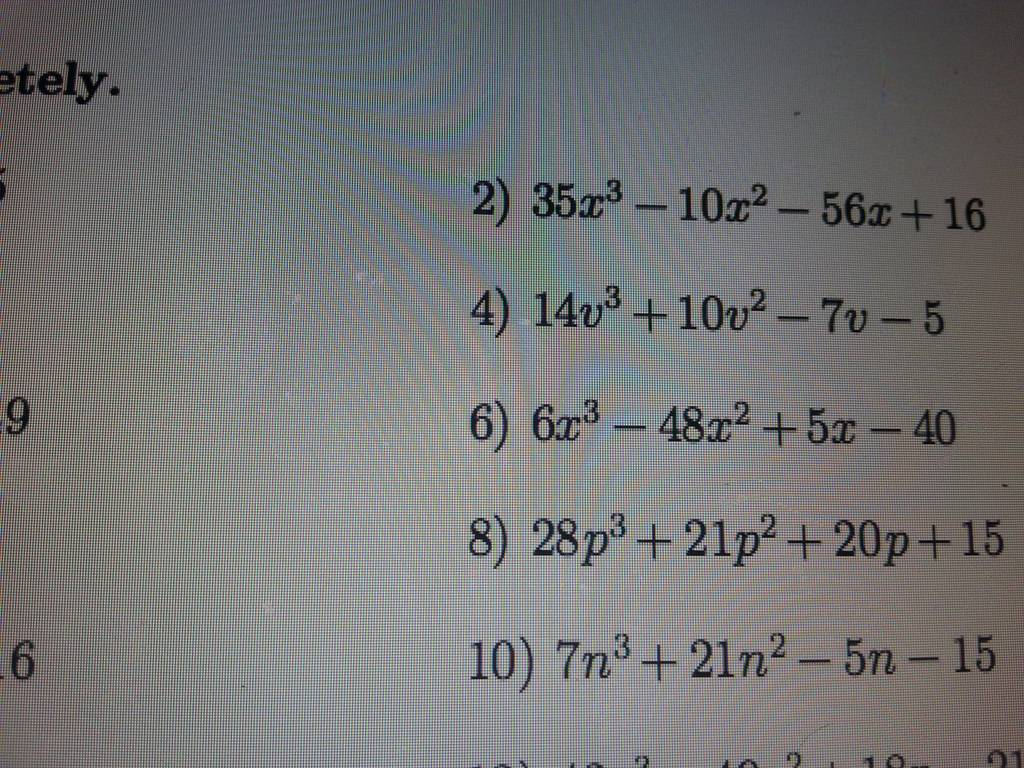# Math Assistance

Math 012

Midterm Exam Page 2

Math 012 Midterm Exam

Spring 2016

Professor:

Name________________________________

Instructions:

· The exam is worth 75 points. There are 15 questions, each worth 5 points. Your score on the exam will be converted to a percentage and posted in your assignment folder with comments.

· This exam is open book and open notes, and you may take as long as you like on it provided that you submit the exam no later than the due date posted in our course schedule of the syllabus. You may refer to your textbook, notes, and online classroom materials, but you may not consult anyone.

· You must show all of your work to receive full credit. If a problem does not seem to require work, write a sentence or two to justify your answer.

· Please type your work in your copy of the exam, or if you prefer, create a document containing your work. Scanned work is also acceptable. Be sure to include your name in the document. Review instructions for submitting your exam in the Exams Module.

At the end of your exam you must include the following dated statement with your name typed in lieu of a signature.  Without this signed statement you will receive a zero.

I have completed this exam myself, working independently and not consulting anyone except the instructor. I have neither given nor received help on this exam.

Name:                                                           Date:

Please remember to show all work on every problem.

1) Solve the equation using the methods discussed in Chapter 1 of our text. If the equation has a unique solution, please show the complete check of your answer.

2) Solve the equation using the methods discussed in Chapter 1 of our text. If the equation has a unique solution, please show the complete check of your answer.

3) Solve the equation using the methods discussed in Chapter 1 of our text. Clear fractions from the equation in the first step. If the equation has a unique solution, please show the complete check of your answer.

4) Solve the inequality using the methods discussed in Chapter 3 of our text. Write your answer in interval notation and graph the solution set on a number line.

5) Solve the inequality using the methods discussed in Chapter 3 of our text. Clear fractions from the inequality in the first step. Write your answer in interval notation and graph the solution set on a number line.

6) Solve the inequality using the methods discussed in Chapter 3 of our text. Write your answer in interval notation and graph the solution set on a number line.

7) Solve the inequality using the methods discussed in Chapter 3 of our text. Write your answer in interval notation and graph the solution set on a number line.

8) The amount of pollution varies directly with the population of a city. City A has a population of 442,000 people and produces 260,000 tons of pollution. How much pollution should we expect City B to produce if its population is 344,000 people? Round your answer to the nearest whole ton.

9) Jeff wins \$600,000 (after taxes) in the lottery and decides to invest half of it in a 10-year CD that pays 7.25% interest compounded monthly. He invests the other half in a money market fund that unfortunately turns out to average only 3.2% interest compounded annually over the 10-year period. How much money will he have altogether in the two accounts at the end of the 10-year period?

10) The average annual tuition and fees at all 4-year institutions in the US in 1982 was \$10,385 and in 2012 was \$ 23,872. Let y be the average tuition and fees in the year x, where x = 0 represents the year 1982.

a) Write a linear equation, in slope-intercept form, that models the growth in average tuition and fees at all 4-year institutions in the US in terms of the year x.

b) Use this equation to predict the average tuition and fees at 4-year institutions in the US in the year 2030.

c) Explain what the slope of this line means in the context of the problem.

11) Given the linear equation :

a) Convert the equation to slope-intercept form. State the slope of the line and the y-intercept as an ordered pair.

b) Use the slope and the y-intercept to graph the line represented by the equation. You may use the axes provided, or create your own graph.12) Given the following two linear equations, determine whether the lines are parallel, perpendicular, or neither. Show all work and explain your conclusion clearly.

13) Write an equation of a line through the point (-5, -2) that is perpendicular to the x-axis. Graph the line on the grid below or create your own graph. State the slope of the line.14) Find an equation of the line through (-6, 10), parallel to the line with equation 3x – 7y = 14. Write the new equation in point-slope form.

15) Convert the equation of the new line found in problem #14 to standard form, Ax + By = C, where A, B, and C are integers.

End of exam: please do not forget to write and sign (or type) the required statement explained on Page 1 of the exam.

(

)

(

)

657612

xxx

-+=-+

2157

153630

aa

-=-

(

)

(

)

343213

mm

->-+

4111

363

xx

-£+

143749

x

-<+£

12516

x

-<-£

5210

xy

-=

-7-6-5-4-3-2-112345678

-7

-6

-5

-4

-3

-2

-1

1

2

3

4

5

6

7

8

x

y

6742

7166

xy

xy

+=

=+

(

)

(

)

4785551

xx

–=-+Basic features
• Free title page and bibliography
• Unlimited revisions
• Plagiarism-free guarantee
• Money-back guarantee